Courses

# Linear Algebra MCQ Level - 2

## 10 Questions MCQ Test Topic wise Tests for IIT JAM Physics | Linear Algebra MCQ Level - 2

Description
This mock test of Linear Algebra MCQ Level - 2 for IIT JAM helps you for every IIT JAM entrance exam. This contains 10 Multiple Choice Questions for IIT JAM Linear Algebra MCQ Level - 2 (mcq) to study with solutions a complete question bank. The solved questions answers in this Linear Algebra MCQ Level - 2 quiz give you a good mix of easy questions and tough questions. IIT JAM students definitely take this Linear Algebra MCQ Level - 2 exercise for a better result in the exam. You can find other Linear Algebra MCQ Level - 2 extra questions, long questions & short questions for IIT JAM on EduRev as well by searching above.
QUESTION: 1

### The system of the equations, x – 4y + 7z = 14 3x + 8y – 2z = 13 7x – 8y + 26z = 5 is :

Solution: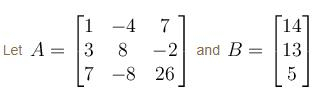Then, consider the augmented matrix,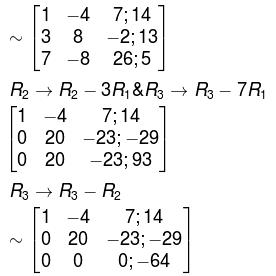∵  Rank A ≠ Rank [A : B]
∴  The system is inconsistent.

QUESTION: 2

### Consider the matrix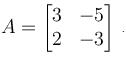Then

Solution: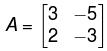tr(A) = 0, |A| = 1
∴ The characteristic polynomial will be,
t(t) = t2 + 1 = 0

Now, A is a matrix over the field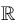.Then t(t) has no real roots. Thus A has no eigenvalues and no eigen vectors.

So, A is not diagonalisable overNow, if A is a matrix over C. Then,

t(t) = t2 – 1
has two roots i and i

Thus, A  has two distinct eigenvalues i and i.

Hence A has two independent eigenvectors. Accordingly, A is diagonalisable over  C.

The correct answer is: A is diagonalisable over C but notQUESTION: 3

### For what values of  k, the given system of equations will have a unique solution? 2x + 3ky + (3k + 4)z = 0 x + (k + 4)y + (4k + 2)z = 0 x + 2(k + 1)y + (3k + 4)z = 0

Solution:

For the unique solution. |A| should be non-zero where A  is the coefficient matrix. i.e.,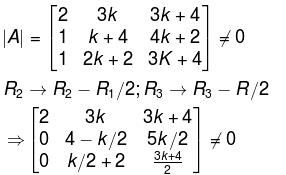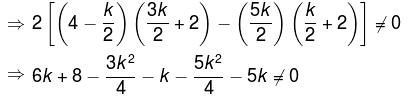⇒ 2k2 + 8 ≠ 0
⇒ k2 ≠ 4
⇒  k ≠ ± 2

The correct answer is: k ≠ ±2

QUESTION: 4

Which of the following will satisfy the given system?
2x1 – 3x2 – 6x3 – 5x4 + 2x5 = 7
x3 + 3x4 – 7x5 = 6
x4 – 2x5 = 1

Solution:

Consider the augmented matrix,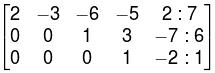We can see that the pivot elements exists in columns 1, 3 and 4

So, x2 and x5  are free variables.

Given, 2x1 – 3x2 – 6x3 – 5x4 + 2x5 = 7     ...(1)
x3 + 3x4 – 7x5 = 6     ...(2)
x4 – 2x5 = 1       ...(3)
from (3),
x4 = 1 + 2x5
from (2),
x3 + 3(1 + 2x5) – 7x5 = 6
x3 = 3 + x5

from (1),
2x1 – 3x2 –6(3 + x5) –5(1 + 2x5) + 2x5 = 7
⇒  2x1 – 3x2 – 18 – 6x5 – 5 - 10x5 + 2x5 = 7
⇒ 2x1 – 3x2 – 23 – 14x5 = 7
⇒ 2x1 = 30 + 3x2 + 14x5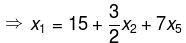∵  x2  and x5 are free variables, Let  x2 = x5 = 0
then  (15, 0, 3, 1, 0)T  becomes one of the solutions.
The correct answer is: (15, 0, 3, 1, 0)T

QUESTION: 5

The system of equations,
x + y + z = 0
2x – y – 3z = 0
3x – 5y + 4z = 0
x + 17y + 4z = 0

Will have :

Solution:

Consider the coefficient matrix,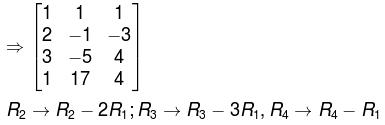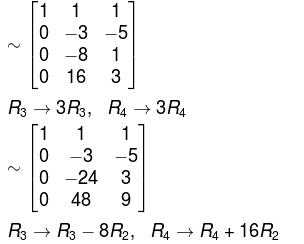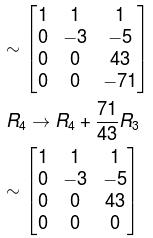Rank of the coefficient matrix is 3 which is equal to the number of unknowns.

The given system of equations possesses non zero solution.

The correct answer is: Unique solution

QUESTION: 6

Choose the correct option about the system,
3x + y + z = 8
x + y – 2z = –5
x + y + z = 6
–2x + 2y – 3z = –7

Solution:

Consider the augmented matrix,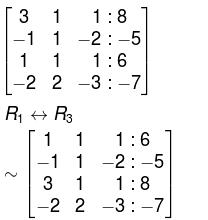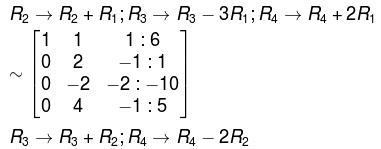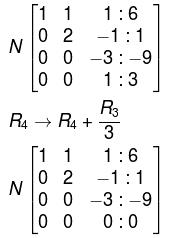∴  Rank of coefficient matrix = Rank of augmented matrix =  Number of unknowns
Hence,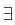unique solution, the system reduces to,
–3z = – 9  ⇒  z = 3
2y – z = 1  ⇒  y = 2
x + y + z = 6  ⇒  x = 1
∴  The solution is  (1, 2, 3)T.

The correct answer is: The system is consistent having unique solution,  (1, 2, 3)T

QUESTION: 7

A necessary and sufficient condition that values, not all zero may be assigned to n variables x1, x2, ......., xn  so that the homogeneous equations, ai1x1 + ai2x2 + ..... + ainxn = 0  (i = 1, 2,.....n) hold simultaneously, is :

Solution:

For the scalars,  ai1, ai2, ..........., ain  (i,= 1, 2,.....,n)
not all zero such that  ai1x1 + ai2x2 + ...... + ain xn = 0
⇔ that  {x1, x2,..........,xn}  is linearly dependent.
⇒ Rank of coefficient matrix will be less than. Hence, minor of order n in the matrix or determinant of the coefficient matrix will be zero.

The correct answer is: |aij|n × n = 0

QUESTION: 8

Consider the following statements about the given system of equations,
x + 2y + 3z  = 0
3x + 4y + 4z  = 0
7x + 10y + 12z  = 0

and choose the correct one

Solution:

Coefficient matrix to the given system of equation is,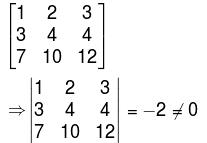Hence, the rank of the coefficient matrix is 3 which is equal to the number of unknown variables in the system.
∴  The system has a unique solution.

The correct answer is: The given system is consistent and has a unique solution.

QUESTION: 9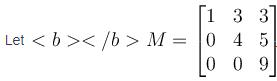Then

Solution:

Since, M  is upper triangular, M2 will also be upper triangular and hence, both of them will be diagonalisable.

The correct answer is: Both M and M2  are diagonalisable

QUESTION: 10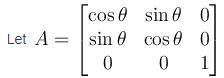Then which of the following option is correct.

Solution:

Determinant  A = cos2 θ +sin2 θ = 1
Hence, A is non-singular and A-1exists

The correct answer is: A is non singular and A–1 exists